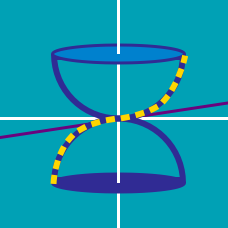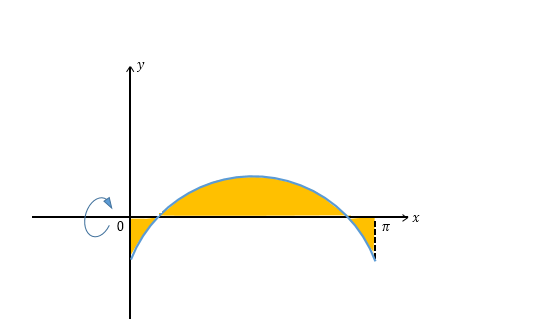Calculus

# Volume of Revolution - Disc Method

Let $V$ be the volume of the solid obtained by revolving the curve $y = x^2$ from $x = 2$ to $3$ about the $x$-axis. What is $10 V?$

Let $V$ be the volume of the solid obtained by revolving the curve $x^2+y^2 -100 =0$ from $x = 0$ to $x=4$ about the $x$-axis. What is $3 V?$

If $a$ is a positive number such that the volume of the solid obtained by rotating the ellipse ${x}^2 + a{y}^2 = 1$ around the $x$-axis is $\frac{4}{57}\pi ,$ what is $a?$The yellow-colored region in the above diagram is bounded by $\begin{array}{c}&y= 18\sin x - a \ (0 < a \ < 18), &x=0, &x=\pi, &y=0 .\end{array}$ What is the value of $a$ that minimizes the volume of the solid obtained by rotating the region around the $x$-axis?

The volume of the solid obtained by rotating the region bounded by $y = x^2 - 2x$ and $y = x$ about the line $y = 6$, has the form $\frac {a}{b} \pi$, where $a$ and $b$ are positive coprime integers. What is the value of $a+b$?

×